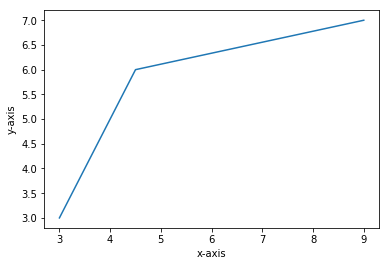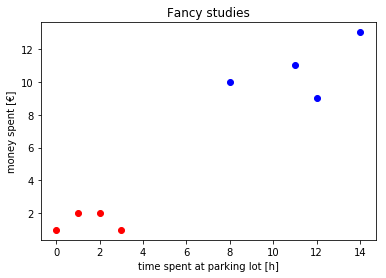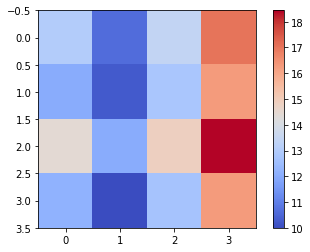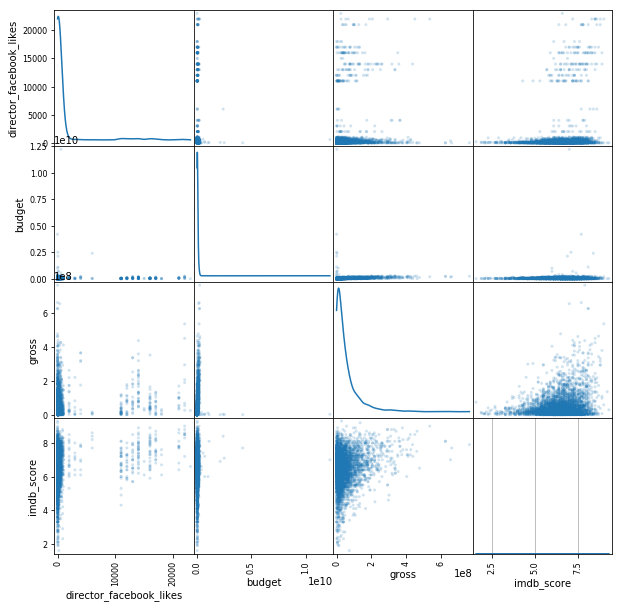Knowledge Discovery and Data Mining I - Winter Semester 2018/19¶

• Lecturer: Prof. Dr. Thomas Seidl
• Assistants: Max Berrendorf, Julian Busch

Tutorial 0: A Short Introduction to Python¶

In this tutorial, we want to give you a short introduction to Python and some insights in the basic usage of some common libraries in the scope of Data Science. The tutorial is intended to prepare you for programming assignments on upcoming exercise sheets. There will be no live coding session in the tutorials, but there will be time for you to ask and discuss questions. The book "Dive Into Python 3" by Mark Pilgrim (http://www.diveintopython3.net/) is a great resource for self-study. If you need any help, feel free to contact your tutor or the assistants.

Installing Python¶

• Anaconda distribution (recommended)

Visit the website https://www.continuum.io/downloads and download the Anaconda distribution for the Python 3.6 version. Make yourself familiar with the jupyter Python notebook which is included in the anaconda installation. If preferred, install also an IDE/editor of your choice, e.g. PyCharm. The Anaconda distribution comes along with a lot of libraries (numpy, scipy, pandas, ...) which otherwise have to be installed individually. If you need an additional library which is not initially included in Anaconda, you can install the lib via

conda install PACKAGENAME.

Further information can be found in the documentation: https://conda.io/docs/index.html

• Without distribution

Visit https://www.python.org/downloads/ and download your preferred python version. Next, go to https://pip.pypa.io/en/stable/installing/ and install $pip$. With $pip$ being installed one can install each package individually. For Example, to install the packages $numpy, scipy, matplotlib, ipython, jupyter, pandas$, execute the following command:

pip install --user numpy scipy matplotlib ipython jupyter pandas

If you need an additional package during the course, don't forget to install the libraries which might be handy.

Basic Python¶

Assigning Values to Variables. Create variables and assign numbers, strings, floating values to them.

In :
prof = "Thomas Seidl"
no_studs = 13
temp = 13.0

print(prof)
Thomas Seidl

Variable Types Python has five standard data types −

• Numbers
• String
• List
• Tuple
• Dictionary

Lists. Create a list which contains all numbers from 0 to 10

In :
l0 = [1,2,3,4,5,6,7,8,9,10]
l0 = list(range(0,10))
l0
Out:
[0, 1, 2, 3, 4, 5, 6, 7, 8, 9]

Loops and Conditionals. Using the created list, print each element of the created list if its is an odd number, by using a loop and conditionals. Try using different type of loops.

In :
#This is a comment
'''
This is a block comment
'''
l1 = [x for x in range(10)]
print(l1)
[0, 1, 2, 3, 4, 5, 6, 7, 8, 9]
In :
# Version with for-loop
for e in l1:
if e%2 != 0:
print(e)
1
3
5
7
9
In :
# Version w/ while-loop
i = 0
while i <len(l1):
# if l1[i] %2 != 0:
#     print(l1[i])
if l1[i] & 1:
print (l1[i])
i+=1
1
3
5
7
9
In :
#Version with list comprehension
l2 = [x for x in l1 if x%2 !=0]
print(l2)
[1, 3, 5, 7, 9]

List Comprehensions. Now, generate a list which contains all numbers from 0 to $n$ which have been squared using list comprehensions.

In :
l3 = [x for x in [x**2 for x in range(7)] if x%2 !=0]
print(l3)
[1, 9, 25]

Functions. Write a function which takes an integer $n$. The function first creates a list of numbers from $0$ to $n$. Then, squares each number of the list. Further each of the squared numbers is tested if it is odd. All odd numbers are then appended to a new list. The function returns the list of odd (squared) numbers.

In :
def get_odd(n):
return [x for x in [x**2 for x in range(n)] if x%2 !=0]

print(get_odd(7))
[1, 9, 25]

Assignments. Given a list $a=['I','like','cookies']$ and another list $b=a$. Replace in the list $b$ the word $'cookies'$ with $'apples'$. Finally, print both lists ($a$ and $b$). What do you observe? What leads to the observed behavior?

In :
b = a

b = 'apples'
print("list a: "+str(a))
print("list b: "+str(b))

print(id(a),id(b))
list a: ['I', 'like', 'apples']
list b: ['I', 'like', 'apples']
139630739802376 139630739802376

Shallow Copy I. Given a list $a=['I','like','cookies']$ and another list which takes a shallow copy of $a$, $b=a[:]$. Like in the previous assignment, replace in the list $b$ the word $'cookies'$ with $'apples'$. Finally, print both lists ($a$ and $b$). What do you observe now?

In :
b3 = a3[:]
b3 = 'apples'
print("list a3: "+str(a3))
print("list b3: "+str(b3))
print(id(a3),id(b3))
print(id(a3),id(b3))
list a3: ['I', 'like', 'cookies']
list b3: ['I', 'like', 'apples']
139630740585480 139630799483848
139630739829568 139630739832536

Shallow Copy II. Now, we are given a list $a = ['I', 'like', ['chocolate', 'cookies']]$. Another list $b = deepcopy(a)$ takes this time a deep copy from $a$. Change now the work $'cookies'$ with $'apples'$ in $b$. Print both lists ($a$ and $b$). What do you observe now?
Hint: For deep copy. first type: from copy import deepcopy

In :
from copy import deepcopy

a4 =  ['I','like',['chocolate', 'cookies']]
b4 = deepcopy(a4)
b4 = 'apples'
print("list a4: "+str(a4))
print("list b4: "+str(b4))
print(id(a4),id(b4))
list a4: ['I', 'like', ['chocolate', 'cookies']]
list b4: ['I', 'like', ['chocolate', 'apples']]
139630739803016 139630799484168

Dictionaries I. Create a dictionary with $n$ entries, where the keys are enumerated from $0$ to $n-1$ and the values are their corresponding keys squared. Use list comprehensions.
Example for expected result: $n = 7; \{0:0, 1:1, 2:4, 3:9, 4:16, 5:25, 6:36\}$

In :
d1 = {x : x**2 for x in range(7)}
print(d1)
{0: 0, 1: 1, 2: 4, 3: 9, 4: 16, 5: 25, 6: 36}

Dictionaries II. Use the dictionary from the previous assignment. Write a list comprehension to get a list of all the keys of the dictionary.

In :
#it actually corresponds to d.keys()
dlis = [d1[x] for x in d1]
print(dlis)
[0, 1, 4, 9, 16, 25, 36]

Lambda Functions. Write a list comprehension which takes a number $n$ and returns a list with even numbers, using a lambda function.

In :
even1 = lambda x: x%2 ==0
l7 = [x for x in range(7) if even1(x)]
print(l7)
[0, 2, 4, 6]

Map. First, write a function which takes a length in $inch$ and returns a length in $cm$. Given a list $l$ with lengths in $inches$: $l=[4,4.5,5,5.5,6,7]$. Write a list comprehension which takes $l$ and returns a list with all values converted to $cm$ using $map()$.

In :
linch = [4,4.5,5,5.5,6,7]

def inch_to_cm(length):
return length*2.54
In :
lcm = list(map(inch_to_cm, linch))
print(lcm)
[10.16, 11.43, 12.7, 13.97, 15.24, 17.78]

Filter. Write a list comprehension which filters the list $l$ from the assignment above by returning only sizes between $4$ and $6$ $inches$.

In :
lrange = list(filter(lambda x: x > 4 and x < 6, linch))
print(lrange)
[4.5, 5, 5.5]

Reduce. Write a list comprehension which reduces the list $l$ by summing up all lenghts.
Hint: for using the reduce function, you need to import it first by: from functools import reduce

In :
from functools import reduce
lsum = reduce(lambda x,y: x+y, linch)
print(lsum)
32.0

List Reverse. Given the following list $a=[0,1,2,3,4,5]$. Write a function which reverses the list.

In :
a = [0,1,2,3,4,5]
a[::-1]
Out:
[5, 4, 3, 2, 1, 0]

Zipping of Lists. Given the following two lists, wher eone list represents the $x-Coordinate$ and another one the $y-Coordinate$:

• $xcoors = [0,1,2,3,4,5]$
• $ycoors = [6,7,8,9,10,11]$

Write a function which zips the two lists to a list of coordinate-tuples:

• $xycoors = [(0,6),(1,7),(2,8),(3,9),(4,10),(5,11)]$
In :
xcoors = [0,1,2,3,4,5]
ycoors = [6,7,8,9,10,11]
zcoors = [99, 98, 97, 96, 95, 94]

#'manual zipping'
def manualzip(lisa, lisb):
reslis = []
for i in range(min(len(lisa),len(lisb))):
reslis.append((lisa[i],lisb[i]))
return reslis

print(manualzip(xcoors,ycoors))

print(list(zip(xcoors,ycoors, zcoors)))
[(0, 6), (1, 7), (2, 8), (3, 9), (4, 10), (5, 11)]
[(0, 6, 99), (1, 7, 98), (2, 8, 97), (3, 9, 96), (4, 10, 95), (5, 11, 94)]

Unzipping of Lists. Now, we are given a list of data points where the first dimension of each data point represents the age of a person and the second dimension the amount of money spent for chocolate per month in euro:

• $chocage = [(20,8), (33,18), (27,14),(66,23),(90,100)]$

Write a function which takes the list and separates it into two lists, one containing the ages and another one containing its corresponding amount of money spent for chocolate. The result would be e.g.:

• $age = [20,33,27,66,90]$
• $money\_spent = [8,18,14,23,100]$
In :
chocage = [(20,8), (33,18), (27,14), (66,23), (90,100)]

#'manual unzipping'
def manualunzip(tuplelis):
lisa = []
lisb = []
for e in tuplelis:
a, b = e
lisa.append(a)
lisb.append(b)
return [tuple(lisa),tuple(lisb)]

print(manualunzip(chocage))

print(list(zip(*chocage)))
[(20, 33, 27, 66, 90), (8, 18, 14, 23, 100)]
[(20, 33, 27, 66, 90), (8, 18, 14, 23, 100)]

Object-oriented Programming¶

Object-oriented Programming I. We deal now with object-oriented programming in Python. For this purpose perform the following steps:

• Write a $Point$ class. A $Point$ class takes and $x$ and $y$ coordinate as an argument.
• Further this class shall have a setter method $setXY$ which takes and $x$ and $y$ coordinate and sets the attributes to the new provided values.
• The class shall also have a getter method $getXY$ which returns the current $x$ and $y$ coordiantes of the point.
• Write a method distance which takes another $point$ object and returns the euclidean distance between the provided point and the point itself. Hint: Take import math to use math.sqrt(value) in order to compute the square root.
In :
import math

class Point(object):

def __init__(self, x, y):
#java: this.x = x;
self.x = x
self.y = y

def setXY(self, x, y):
self.x = x
self.y = y

def getXY(self):
return (self.x,self.y)

def distance(self, otherpoint):
d = (self.x-otherpoint.x)**2 + (self.y-otherpoint.y)**2
return math.sqrt(d)

Object-oriented Programming II. In a next step, the task is to create a class $Shape$. For this purpose perform the following steps:

• Create a class $Shape$ which takes a name and a color as parameters.
• Define a method $area$ which just returns $0.0$.
• Define a method $perimeter$ which just return $0.0$.

Now, create a class Rectangle which inherits from $Shape$ and in which you $implement$ the $area$ and $perimeter$ methods.

In :
class Shape(object):

def __init__(self, name, color):
self.name = name
self.color = color

def area(self):
return 0.0

def perimeter(self):
return 0.0

class Rectangle(Shape):
def __init__(self, corner, width, height, color):
#super(...) 'equivalent':
Shape.__init__(self, "rectangle", color)
self.corner = corner
self.width = width
self.height = height

def perimeter(self):
return self.width*2 + self.height*2

def area(self):
return self.width * self.height

r = Rectangle(Point(4,4),10,5,'pink')
print('Perimeter of rectangle r: ',r.perimeter())
print('Area of rectangle r: ', r.area())

Perimeter of rectangle r:  30
Area of rectangle r:  50

Numpy¶

Numpy I - Some Basic Functions. In this block, you will become familiar with the numpy library and some of its basic functionality. Please also consider to consult the documentation https://docs.scipy.org/doc/numpy-dev/index.html if needed. Solve the following tasks:

• Create an numpy array of floats containing the numbers from $0$ to $4$.
• Create the following matrix as a numpy matrix: $M = [[1,2,3], [4,5,6]]$.
• Get the shape of the matrix $M$.
• Check if the value $2$ is in $M$.
• Given the array $a = np.array([0,1,2,3,4,5,6,7,8,9], float)$. Reshape it to an $5\times2$ matrix.
• Transpose the previously introduced matrix $M$.
• Flatten matrix $M$.
• Given the array $b = np.array ([0,1,2,3], float)$. Increase the dimensionality of $b$.
• Create and $3\times3$ identity matrix.
In :
import numpy as np

#create an np array with float as type
arr0 = np.array([1,2,3,4], float)
arr0

#create a 2x3 matrix using np arrays
arr1 = np.array([[1,2,3],[4,5,6]], float)
arr1[0,0]

#get shape of an array
arr1.shape

#getting type of array
arr1.dtype

#check if a particular value is in the array
[1,2,3] in arr1

#reshape an array e.g. 1x10 to an 5x2 array
arr2 = np.array(range(10), float)
#print(arr2)
arr3 = arr2.reshape((5,2))
#print(arr3)

#fill matrix with specific value
arr4 = np.array(range(10))
arr4.fill(42)
print(arr4)

#transpose an array
arr5 = np.array([[1,2,3],[4,5,6]], float)
arr6 = arr5.transpose()
print(arr5)
print(arr6)

#flatten an array...
print(arr6.flatten())

#increasing dimensionality of an array
arr7 = np.array([1,2,3],float)
print(arr7)
print(arr7[:,np.newaxis])

#array of ones and zeros
print("array of ones and zeros")
print(np.ones((2,3),float))
print(np.zeros((2,3),float))

#getting an identity matrix
print(np.identity(3,float))
[42 42 42 42 42 42 42 42 42 42]
[[1. 2. 3.]
[4. 5. 6.]]
[[1. 4.]
[2. 5.]
[3. 6.]]
[1. 4. 2. 5. 3. 6.]
[1. 2. 3.]
[[1.]
[2.]
[3.]]
array of ones and zeros
[[1. 1. 1.]
[1. 1. 1.]]
[[0. 0. 0.]
[0. 0. 0.]]
[[1. 0. 0.]
[0. 1. 0.]
[0. 0. 1.]]

Numpy II - Linear Algebra and Statistics. This assignemtn has its focus on numpy function of the linear algebra and statistics domain. Solve the following tasks using numpy:

• Given the following two numpy array: $a=np.array([1,2,3], float)$, $b=([4,5,6],float)$. Compute the dot product of $a$ and $b$
• Given the following matrix $M = [[1,2,3], [4,5,6], [7,8,9]]$, compute the determinant of $M$ by using the $linalg$ package of the numpy library.
• Compute the eigenvalues and eigenvectors of $M$
• Compute the inverse of $M$
• Given the numpy array $c=np.array([1,4,3,8,3,2,3], float)$, compute the mean of $c$
• using $c$, compute the median.
• given the following matrix $C=[[1,1], [3,4]]$, compute the covariance of $C$.
In :
# DOT PRODUCT
arr8 = np.array([1,2,3],float)
arr9 = np.array([4,5,6],float)
print(np.dot(arr8,arr9))

# DETERMINANT
arr10 = np.array([[1,2,3],[4,5,6],[7,8,9]],float)
print(np.linalg.det(arr10))

# COMPUTE EIGENVALUES AND EIGENVECTORS
eigenvals, eigenvecs = np.linalg.eig(arr10)
print(eigenvals)
print("------")
print(eigenvecs)

# COMPUTE INVERSE
print(np.linalg.inv(arr10))

# COMPUTE MEAN AND MEDIAN
arr11 = np.array([1,4,3,8,9,2,3],float)
print("mean: ",np.mean(arr11))
print("median: ",np.median(arr11))

# COMPUTE COVARIANCE
arr12 = np.array([[1,1],[3,4]],float)
print('cov: ',np.cov(arr12))
32.0
6.66133814775094e-16
[ 1.61168440e+01 -1.11684397e+00 -1.30367773e-15]
------
[[-0.23197069 -0.78583024  0.40824829]
[-0.52532209 -0.08675134 -0.81649658]
[-0.8186735   0.61232756  0.40824829]]
[[-4.50359963e+15  9.00719925e+15 -4.50359963e+15]
[ 9.00719925e+15 -1.80143985e+16  9.00719925e+15]
[-4.50359963e+15  9.00719925e+15 -4.50359963e+15]]
mean:  4.285714285714286
median:  3.0
cov:  [[0.  0. ]
[0.  0.5]]

Matplotlib¶

Matplotlib - Plotting Figures in Python. In this assignment we are finally going to become familiar with the plotting library of Python. For this we solve the following tasks below. Please consider to consult the documentation if needed: https://matplotlib.org/contents.html.

• Given a list of data points : $dpts=[(3,3),(4,5),(4.5,6),(9,7)]$. Plot the function using $plt.plot(xcoors, ycoors)$
• You are given two tiny clusters $c_1 = [(1,2),(3,1),(0,1),(2,2)]$ and $c_2=[(12,9),(8,10),(11,11), (14,13)]$. Plot them in a scatter plot using $plt.scatter(xcoors, ycoors)$, where $c_1$ and $c_2$ have different colors. The $x-axis$ represents the time spent at a parking lot in hours, and the $y-axis$ represents the money spent in euro. Create axis labels for your figure.
• Take the two clusters $c_1$ and $c_2$ together and compute their pairwise distances, storing them in a matrix. Plot the resulting matrix as a heatmap using $plt.imshow(my\_matrix, cmap='coolwarm')$.
In :
import matplotlib.pyplot as plt
%matplotlib inline

#1
dpts = np.asarray([(3,3),(4,5),(4.5,6),(9,7)])
#access second column (y-coordinates)
print(dpts[:,1])

plt.figure()
plt.plot(dpts[:,0],dpts[:,1])
plt.ylabel('y-axis')
plt.xlabel('x-axis')
plt.show()

#2 scatter plot
c1 = np.array([(1,2),(3,1),(0,1),(2,2)])
c2 = np.array([(12,9),(8,10),(11,11),(14,13)])

plt.figure()
plt.scatter(c1[:,0],c1[:,1], color='r')
plt.scatter(c2[:,0],c2[:,1], color='b')
plt.xlabel('time spent at parking lot [h]')
plt.ylabel('money spent [€]')
plt.title("Fancy studies")
plt.show()

#3 now for something completely different: heatmap...
from scipy.spatial import distance

distmx = []
for e in c1:
newrow = []
for f in c2:
d = distance.euclidean(e,f)
newrow.append(d)
distmx.append(newrow)

for e in distmx:
print(e)

plt.imshow(distmx, cmap='coolwarm', interpolation='nearest')
plt.colorbar()
plt.show()
[3. 5. 6. 7.][13.038404810405298, 10.63014581273465, 13.45362404707371, 17.029386365926403]
[12.041594578792296, 10.295630140987, 12.806248474865697, 16.278820596099706]
[14.422205101855956, 12.041594578792296, 14.866068747318506, 18.439088914585774]
[12.206555615733702, 10.0, 12.727922061357855, 16.278820596099706]Pandas¶

Pandas- Basic Data Analysis. For this assignment, we will use the file moviemetadata.csv, which contains entries from the IMDB movie database. The original source of the data is Kaggle: https://www.kaggle.com/deepmatrix/imdb-5000-movie-dataset/. Please also consider to consult the documentation http://pandas.pydata.org/pandas-docs/stable/ if needed. Solve the following tasks:

• Read the csv file as a DataFrame for further processing using $pandas.read csv()$.
• Inspect the read csv file using $.shape$, $.columns$, $.info()$ and $.describe()$.
• Display the first five records of the data set using $.head(5)$ and the last five records using $.tail(5)$.
• Select from the data set the first five records. Those records shall only contain the following columns: $movie\_title$, $duration$ and $num\_voted\_users$.
• Select the first five movies containing the genre $Action$. Display only the columns $movie\_title$ and $genres$.
• Sort the action movies by their $imdb\_score$ and display the names and scores the top-10 scored movies.
• Group the movies by column $director$ and display the top-10 directors with the highest mean gross of their movies.
• Optional: Delete all rows, which contain at least one missing value. Visualize parts of the data using $pandas.plotting.scatter\_matrix$ and $groupby.DataFrameGroupBy.hist$.
In :
import pandas as pd
from pandas.plotting import scatter_matrix
import matplotlib.pyplot as plt
plt.style.use('default')
%matplotlib inline

# Read movie dataset
delimiter=',',
decimal='.')

# Get an overview
print('== OVERVIEW ==')
print(movie_data.shape)
print(movie_data.columns)
movie_data.info()
display(movie_data.describe())

# Show first/last 5 records
display(movie_data.tail(5))

# Indexing
print('== INDEXING ==')

# Filtering
print('== FILTERING ==')

# Sorting
print('== SORTING ==')

# Grouping
print('== GROUPING ==')

# Delete rows with NaNs
print('== DELETION OF NAN ROWS ==')
print(movie_data.shape)
movie_data = movie_data.dropna(axis=0, how='any')
print(movie_data.shape)

# Visualization
print('== VISUALIZATION ==')
scatter_matrix(movie_data[['director_facebook_likes', 'budget', 'gross', 'imdb_score']], alpha=0.2, figsize=(10, 10), diagonal='kde')
movie_data.groupby('color')['title_year'].hist(alpha=0.4, figsize=(10, 10))
== OVERVIEW ==
(5043, 28)
Index(['color', 'director_name', 'num_critic_for_reviews', 'duration',
'actor_1_facebook_likes', 'gross', 'genres', 'actor_1_name',
'actor_3_name', 'facenumber_in_poster', 'plot_keywords',
'movie_imdb_link', 'num_user_for_reviews', 'language', 'country',
'content_rating', 'budget', 'title_year', 'actor_2_facebook_likes',
dtype='object')
<class 'pandas.core.frame.DataFrame'>
RangeIndex: 5043 entries, 0 to 5042
Data columns (total 28 columns):
color                        5024 non-null object
director_name                4939 non-null object
num_critic_for_reviews       4993 non-null float64
duration                     5028 non-null float64
director_facebook_likes      4939 non-null float64
actor_3_facebook_likes       5020 non-null float64
actor_2_name                 5030 non-null object
actor_1_facebook_likes       5036 non-null float64
gross                        4159 non-null float64
genres                       5043 non-null object
actor_1_name                 5036 non-null object
movie_title                  5043 non-null object
num_voted_users              5043 non-null int64
cast_total_facebook_likes    5043 non-null int64
actor_3_name                 5020 non-null object
facenumber_in_poster         5030 non-null float64
plot_keywords                4890 non-null object
movie_imdb_link              5043 non-null object
num_user_for_reviews         5022 non-null float64
language                     5031 non-null object
country                      5038 non-null object
content_rating               4740 non-null object
budget                       4551 non-null float64
title_year                   4935 non-null float64
actor_2_facebook_likes       5030 non-null float64
imdb_score                   5043 non-null float64
aspect_ratio                 4714 non-null float64
movie_facebook_likes         5043 non-null int64
dtypes: float64(13), int64(3), object(12)
memory usage: 1.1+ MB
count 4993.000000 5028.000000 4939.000000 5020.000000 5036.000000 4.159000e+03 5.043000e+03 5043.000000 5030.000000 5022.000000 4.551000e+03 4935.000000 5030.000000 5043.000000 4714.000000 5043.000000
mean 140.194272 107.201074 686.509212 645.009761 6560.047061 4.846841e+07 8.366816e+04 9699.063851 1.371173 272.770808 3.975262e+07 2002.470517 1651.754473 6.442138 2.220403 7525.964505
std 121.601675 25.197441 2813.328607 1665.041728 15020.759120 6.845299e+07 1.384853e+05 18163.799124 2.013576 377.982886 2.061149e+08 12.474599 4042.438863 1.125116 1.385113 19320.445110
min 1.000000 7.000000 0.000000 0.000000 0.000000 1.620000e+02 5.000000e+00 0.000000 0.000000 1.000000 2.180000e+02 1916.000000 0.000000 1.600000 1.180000 0.000000
25% 50.000000 93.000000 7.000000 133.000000 614.000000 5.340988e+06 8.593500e+03 1411.000000 0.000000 65.000000 6.000000e+06 1999.000000 281.000000 5.800000 1.850000 0.000000
50% 110.000000 103.000000 49.000000 371.500000 988.000000 2.551750e+07 3.435900e+04 3090.000000 1.000000 156.000000 2.000000e+07 2005.000000 595.000000 6.600000 2.350000 166.000000
75% 195.000000 118.000000 194.500000 636.000000 11000.000000 6.230944e+07 9.630900e+04 13756.500000 2.000000 326.000000 4.500000e+07 2011.000000 918.000000 7.200000 2.350000 3000.000000
max 813.000000 511.000000 23000.000000 23000.000000 640000.000000 7.605058e+08 1.689764e+06 656730.000000 43.000000 5060.000000 1.221550e+10 2016.000000 137000.000000 9.500000 16.000000 349000.000000
color director_name num_critic_for_reviews duration director_facebook_likes actor_3_facebook_likes actor_2_name actor_1_facebook_likes gross genres ... num_user_for_reviews language country content_rating budget title_year actor_2_facebook_likes imdb_score aspect_ratio movie_facebook_likes
0 Color James Cameron 723.0 178.0 0.0 855.0 Joel David Moore 1000.0 760505847.0 Action|Adventure|Fantasy|Sci-Fi ... 3054.0 English USA PG-13 237000000.0 2009.0 936.0 7.9 1.78 33000
1 Color Gore Verbinski 302.0 169.0 563.0 1000.0 Orlando Bloom 40000.0 309404152.0 Action|Adventure|Fantasy ... 1238.0 English USA PG-13 300000000.0 2007.0 5000.0 7.1 2.35 0
2 Color Sam Mendes 602.0 148.0 0.0 161.0 Rory Kinnear 11000.0 200074175.0 Action|Adventure|Thriller ... 994.0 English UK PG-13 245000000.0 2015.0 393.0 6.8 2.35 85000
3 Color Christopher Nolan 813.0 164.0 22000.0 23000.0 Christian Bale 27000.0 448130642.0 Action|Thriller ... 2701.0 English USA PG-13 250000000.0 2012.0 23000.0 8.5 2.35 164000
4 NaN Doug Walker NaN NaN 131.0 NaN Rob Walker 131.0 NaN Documentary ... NaN NaN NaN NaN NaN NaN 12.0 7.1 NaN 0

5 rows × 28 columns

color director_name num_critic_for_reviews duration director_facebook_likes actor_3_facebook_likes actor_2_name actor_1_facebook_likes gross genres ... num_user_for_reviews language country content_rating budget title_year actor_2_facebook_likes imdb_score aspect_ratio movie_facebook_likes
5038 Color Scott Smith 1.0 87.0 2.0 318.0 Daphne Zuniga 637.0 NaN Comedy|Drama ... 6.0 English Canada NaN NaN 2013.0 470.0 7.7 NaN 84
5039 Color NaN 43.0 43.0 NaN 319.0 Valorie Curry 841.0 NaN Crime|Drama|Mystery|Thriller ... 359.0 English USA TV-14 NaN NaN 593.0 7.5 16.00 32000
5040 Color Benjamin Roberds 13.0 76.0 0.0 0.0 Maxwell Moody 0.0 NaN Drama|Horror|Thriller ... 3.0 English USA NaN 1400.0 2013.0 0.0 6.3 NaN 16
5041 Color Daniel Hsia 14.0 100.0 0.0 489.0 Daniel Henney 946.0 10443.0 Comedy|Drama|Romance ... 9.0 English USA PG-13 NaN 2012.0 719.0 6.3 2.35 660
5042 Color Jon Gunn 43.0 90.0 16.0 16.0 Brian Herzlinger 86.0 85222.0 Documentary ... 84.0 English USA PG 1100.0 2004.0 23.0 6.6 1.85 456

5 rows × 28 columns

== INDEXING ==
movie_title duration num_voted_users
0 Avatar 178.0 886204
1 Pirates of the Caribbean: At World's End 169.0 471220
2 Spectre 148.0 275868
3 The Dark Knight Rises 164.0 1144337
4 Star Wars: Episode VII - The Force Awakens  ... NaN 8
== FILTERING ==
movie_title genres
1 Pirates of the Caribbean: At World's End Action|Adventure|Fantasy
3 The Dark Knight Rises Action|Thriller
5 John Carter Action|Adventure|Sci-Fi
== SORTING ==
movie_title imdb_score
4409 Kickboxer: Vengeance 9.1
66 The Dark Knight 9.0
339 The Lord of the Rings: The Return of the King 8.9
270 The Lord of the Rings: The Fellowship of the R... 8.8
459 Daredevil 8.8
2051 Star Wars: Episode V - The Empire Strikes Back 8.8
97 Inception 8.8
4468 Queen of the Mountains 8.7
654 The Matrix 8.7
340 The Lord of the Rings: The Two Towers 8.7
== GROUPING ==
director_name
Joss Whedon        4.327217e+08
Lee Unkrich        4.149845e+08
Chris Buck         4.007366e+08
Tim Miller         3.630243e+08
George Lucas       3.482837e+08
Kyle Balda         3.360296e+08
Colin Trevorrow    3.280925e+08
Yarrow Cheney      3.235055e+08
Pete Docter        3.131138e+08
Pierre Coffin      3.097756e+08
Name: gross, dtype: float64
== DELETION OF NAN ROWS ==
(5043, 28)
(3756, 28)
== VISUALIZATION ==
Out:
color
Black and White    AxesSubplot(0.70625,0.125;0.19375x0.18875)
Color               AxesSubplot(0.70625,0.125;0.19375x0.18875)
Name: title_year, dtype: object